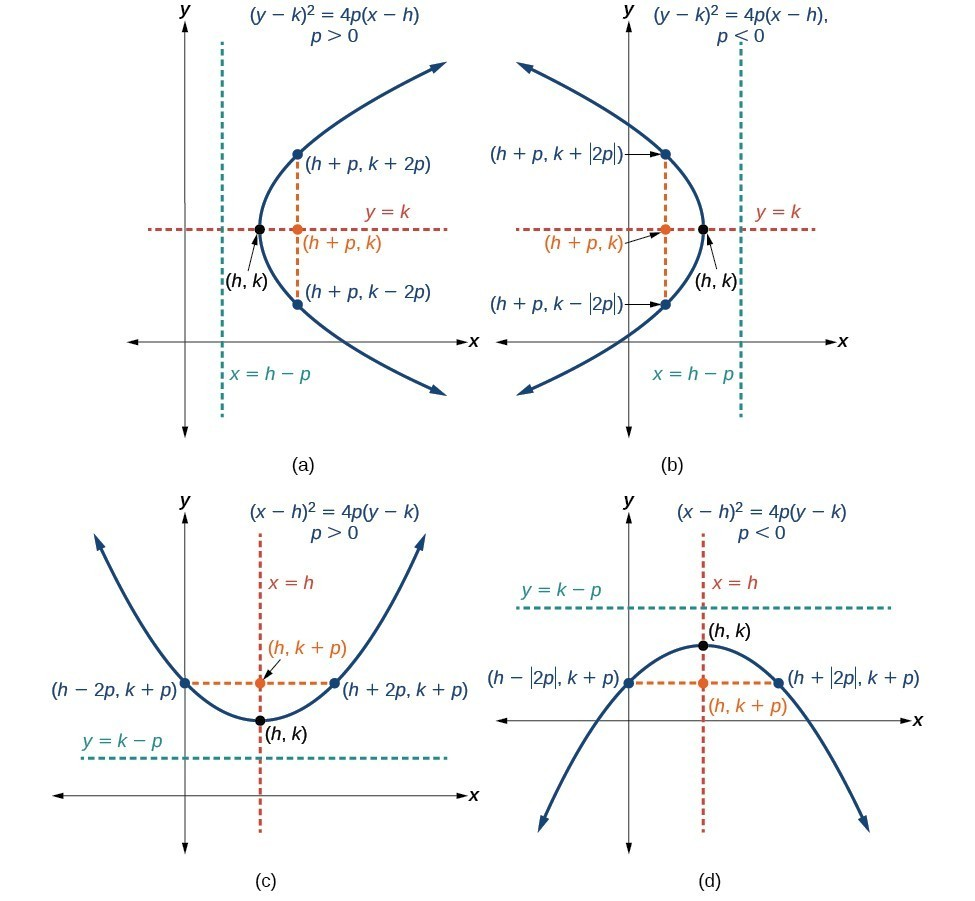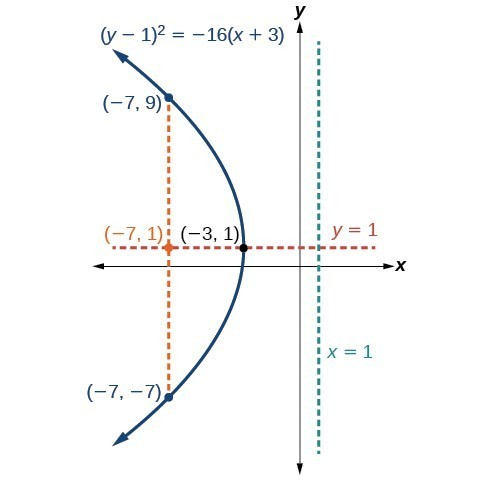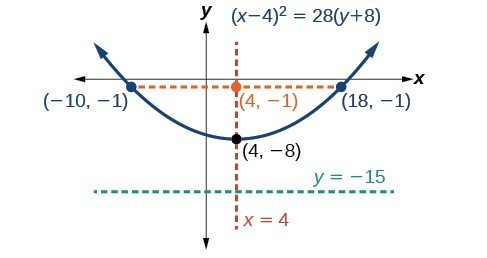## Graphing Parabolas with Vertices Not at the Origin

Like other graphs we’ve worked with, the graph of a parabola can be translated. If a parabola is translated $h$ units horizontally and $k$ units vertically, the vertex will be $\left(h,k\right)$. This translation results in the standard form of the equation we saw previously with $x$ replaced by $\left(x-h\right)$ and $y$ replaced by $\left(y-k\right)$.

To graph parabolas with a vertex $\left(h,k\right)$ other than the origin, we use the standard form ${\left(y-k\right)}^{2}=4p\left(x-h\right)$ for parabolas that have an axis of symmetry parallel to the x-axis, and ${\left(x-h\right)}^{2}=4p\left(y-k\right)$ for parabolas that have an axis of symmetry parallel to the y-axis. These standard forms are given below, along with their general graphs and key features.

### A General Note: Standard Forms of Parabolas with Vertex (h, k)

The table and Figure 9 summarize the standard features of parabolas with a vertex at a point $\left(h,k\right)$.

 Axis of Symmetry Equation Focus Directrix Endpoints of Latus Rectum $y=k$ ${\left(y-k\right)}^{2}=4p\left(x-h\right)$ $\left(h+p,\text{ }k\right)$ $x=h-p$ $\left(h+p,\text{ }k\pm 2p\right)$ $x=h$ ${\left(x-h\right)}^{2}=4p\left(y-k\right)$ $\left(h,\text{ }k+p\right)$ $y=k-p$ $\left(h\pm 2p,\text{ }k+p\right)$Figure 9. (a) When $p>0$, the parabola opens right. (b) When $p<0$, the parabola opens left. (c) When $p>0$, the parabola opens up. (d) When $p<0$, the parabola opens down.

### How To: Given a standard form equation for a parabola centered at (h, k), sketch the graph.

1. Determine which of the standard forms applies to the given equation: ${\left(y-k\right)}^{2}=4p\left(x-h\right)$ or ${\left(x-h\right)}^{2}=4p\left(y-k\right)$.
2. Use the standard form identified in Step 1 to determine the vertex, axis of symmetry, focus, equation of the directrix, and endpoints of the latus rectum.
1. If the equation is in the form ${\left(y-k\right)}^{2}=4p\left(x-h\right)$, then:
• use the given equation to identify $h$ and $k$ for the vertex, $\left(h,k\right)$
• use the value of $k$ to determine the axis of symmetry, $y=k$
• set $4p$ equal to the coefficient of $\left(x-h\right)$ in the given equation to solve for $p$. If $p>0$, the parabola opens right. If $p<0$, the parabola opens left.
• use $h,k$, and $p$ to find the coordinates of the focus, $\left(h+p,\text{ }k\right)$
• use $h$ and $p$ to find the equation of the directrix, $x=h-p$
• use $h,k$, and $p$ to find the endpoints of the latus rectum, $\left(h+p,k\pm 2p\right)$
2. If the equation is in the form ${\left(x-h\right)}^{2}=4p\left(y-k\right)$, then:
• use the given equation to identify $h$ and $k$ for the vertex, $\left(h,k\right)$
• use the value of $h$ to determine the axis of symmetry, $x=h$
• set $4p$ equal to the coefficient of $\left(y-k\right)$ in the given equation to solve for $p$. If $p>0$, the parabola opens up. If $p<0$, the parabola opens down.
• use $h,k$, and $p$ to find the coordinates of the focus, $\left(h,\text{ }k+p\right)$
• use $k$ and $p$ to find the equation of the directrix, $y=k-p$
• use $h,k$, and $p$ to find the endpoints of the latus rectum, $\left(h\pm 2p,\text{ }k+p\right)$
3. Plot the vertex, axis of symmetry, focus, directrix, and latus rectum, and draw a smooth curve to form the parabola.

### Example 5: Graphing a Parabola with Vertex (h, k) and Axis of Symmetry Parallel to the x-axis

Graph ${\left(y - 1\right)}^{2}=-16\left(x+3\right)$. Identify and label the vertex, axis of symmetry, focus, directrix, and endpoints of the latus rectum.

### Solution

The standard form that applies to the given equation is ${\left(y-k\right)}^{2}=4p\left(x-h\right)$. Thus, the axis of symmetry is parallel to the x-axis. It follows that:

• the vertex is $\left(h,k\right)=\left(-3,1\right)$
• the axis of symmetry is $y=k=1$
• $-16=4p$, so $p=-4$. Since $p<0$, the parabola opens left.
• the coordinates of the focus are $\left(h+p,k\right)=\left(-3+\left(-4\right),1\right)=\left(-7,1\right)$
• the equation of the directrix is $x=h-p=-3-\left(-4\right)=1$
• the endpoints of the latus rectum are $\left(h+p,k\pm 2p\right)=\left(-3+\left(-4\right),1\pm 2\left(-4\right)\right)$, or $\left(-7,-7\right)$ and $\left(-7,9\right)$

Next we plot the vertex, axis of symmetry, focus, directrix, and latus rectum, and draw a smooth curve to form the parabola.Figure 10

### Try It 5

Graph ${\left(y+1\right)}^{2}=4\left(x - 8\right)$. Identify and label the vertex, axis of symmetry, focus, directrix, and endpoints of the latus rectum.

Solution

### Example 6: Graphing a Parabola from an Equation Given in General Form

Graph ${x}^{2}-8x - 28y - 208=0$. Identify and label the vertex, axis of symmetry, focus, directrix, and endpoints of the latus rectum.

### Solution

Start by writing the equation of the parabola in standard form. The standard form that applies to the given equation is ${\left(x-h\right)}^{2}=4p\left(y-k\right)$. Thus, the axis of symmetry is parallel to the y-axis. To express the equation of the parabola in this form, we begin by isolating the terms that contain the variable $x$ in order to complete the square.

$\begin{array}{l}{x}^{2}-8x - 28y - 208=0\hfill \\ \text{ }{x}^{2}-8x=28y+208\hfill \\ \text{ }{x}^{2}-8x+16=28y+208+16\hfill \\ \text{ }{\left(x - 4\right)}^{2}=28y+224\hfill \\ \text{ }{\left(x - 4\right)}^{2}=28\left(y+8\right)\hfill \\ \text{ }{\left(x - 4\right)}^{2}=4\cdot 7\cdot \left(y+8\right)\hfill \end{array}$

It follows that:

• the vertex is $\left(h,k\right)=\left(4,-8\right)$
• the axis of symmetry is $x=h=4$
• since $p=7,p>0$ and so the parabola opens up
• the coordinates of the focus are $\left(h,k+p\right)=\left(4,-8+7\right)=\left(4,-1\right)$
• the equation of the directrix is $y=k-p=-8 - 7=-15$
• the endpoints of the latus rectum are $\left(h\pm 2p,k+p\right)=\left(4\pm 2\left(7\right),-8+7\right)$, or $\left(-10,-1\right)$ and $\left(18,-1\right)$

Next we plot the vertex, axis of symmetry, focus, directrix, and latus rectum, and draw a smooth curve to form the parabola.Figure 11

### Try It 6

Graph ${\left(x+2\right)}^{2}=-20\left(y - 3\right)$. Identify and label the vertex, axis of symmetry, focus, directrix, and endpoints of the latus rectum.

Solution